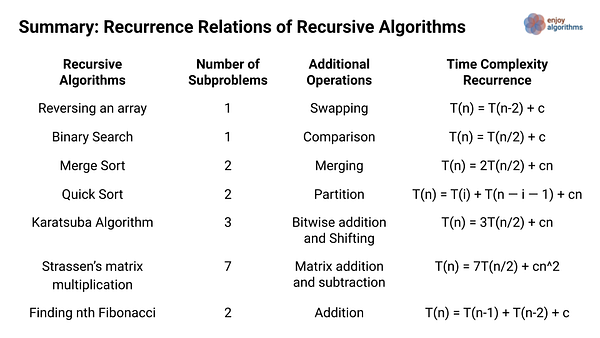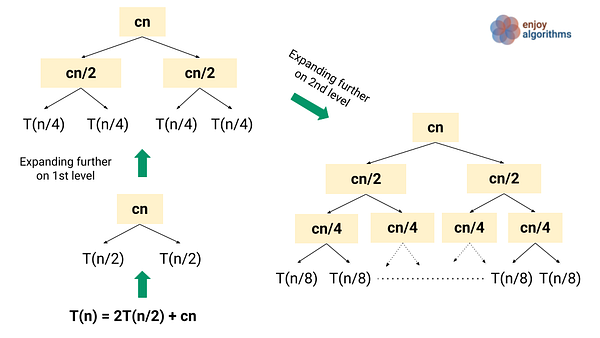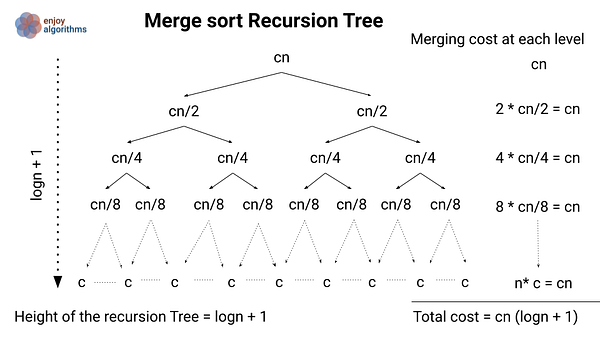# Analysis of Recursion in Data Structure and Algorithms

Recursion is one of the popular problem-solving approaches in data structure and algorithms. Even some problem-solving approaches are totally based on recursion: decrease and conquer, divide and conquer, DFS traversal of tree and graph, backtracking, top-down approach of dynamic programming, etc. So learning time complexity analysis of recursion is critical to understand these approaches and improve the efficiency of our code.

Sometimes programmers find challenging to analyze recursion due to the mathematical details behind it. But working with recursive algorithms becomes easy when we have a good understanding of recursive patterns and methods used in the analysis. Without delaying further, let's learn fundamental concepts related to the analysis of recursion:

### What is recurrence relation in algorithms?

A recurrence relation is an equation that describes a sequence where any term is defined in terms of its previous terms. We use recurrence relation to define and analyse the time complexity of recursive algorithms in terms of input size.

In the case of recursion, we solve the problem using the solution of the smaller subproblems. If the time complexity function of the algorithm is T(n), then the time complexity of the smaller sub-problems will be defined by the same function but in terms of the input size of the subproblems. Here is a common approach to write T(n) if we have k number of subproblems:

T(n) = T(input size of subproblem 1) + T(input size of subproblem 2)....+ T(input size of subproblem k) + Time complexity of additional operations other than recursive calls

The above formula provides an approach to define the recurrence relation of every recursive algorithm. Then, we solve this recurrence relation and calculate the overall time complexity in terms of Big-O notation. Let's understand this better via the examples of various recurrence relations of popular recursive algorithms.

Decrease by a constant: Reverse an array

``````reverse (A[], l, r)
- swap(A[l], A[r])
- reverse(A, l + 1, r - 1)

Base Case: if (l >= r) then return
``````

We are reversing the n size array by swapping the first & last value and the recursively solving remaining subproblem of the (n - 2) size array. At each step of recursion, the input size is decreasing by 2.

• Time complexity T(n) = Time complexity of solving (n - 2) size problem + Time Complexity of one swap operation = T(n - 2) + O(1)
• Recurrence relation of reverse an array: T(n) = T(n - 2) + c, where T(1) = c

Decrease by a constant factor: Binary search

``````binarySearch(A[], l, r, k)
- if A[mid] = k, return mid
- if (A[mid] > k), binarySearch(A[], l, mid-1, k)
- if (A[mid] < k), binarySearch(A[], mid+1, r, k)

Base Case: If (l > r) then return -1
``````

At each step of recursion, we are doing one comparison and decreasing the input size by half. In other words, we are solving the problem of n size by the solution of one subproblem of input size n/2 (Based on the comparison, either left or right sub-problem)

• Time complexity T(n) = Time complexity of n/2 size problem + Time Complexity of comparison operation = T(n/2) + O(1).
• Recurrence Relation of binary search:T(n) = T(n/2) + c, where T(1) = c

Dividing into two equal size subproblems: Merge sort

``````mergeSort (A[], l, r)
- mid = l + (r - l)/2
- mergeSort(A, l, mid)
- mergeSort(A, mid + 1, r)
- merge(A, l, mid, r)

Base Case: if (l == r) then return
``````

In merge sort, we solve the problem of n size by the solution of two equal subproblems of input size n/2 and merging the solution in O(n) time complexity.

• Time complexity T(n) = Time complexity of left sub-problem of size n/2 + Time complexity of right sub-problem of size n/2 + Time Complexity of merging = T(n/2) + T(n/2) + O(n) = 2 T(n/2) + cn
• Recurrence Relation of merge sort: T(n) = 2T(n/2) + cn, where T(1) = c

Dividing into two different size subproblems: Quick sort

``````quickSort(A[], l, r)
- pivot = partition(A, l, r)
- quickSort(A, l, pivot - 1)
- quickSort(A, pivot + 1, r)

Base Case: if (l >= r) then return
``````

In quick sort, we are dividing the array in O(n) time and recursively solving two subproblems of different sizes. The size of the subproblems depends on the choice of the pivot in the partition algorithm. Suppose after the partition, i elements are in the left subarray (left of the pivot), and n-i-1 elements are in the right subarray (right of the pivot). Size of the left subproblem = i, size of the right subproblem = n — i — 1.

• So time complexity T(n) = Time complexity of partition algorithm + Time complexity of left sub-problem of size i + Time complexity of right sub-problem of size (n - i - 1) = O(n) + T(i) + T(n-i-1) = T(i) + T(n — i — 1) + cn
• Recurrence relation of quick sort: T(n) = T(i) + T(n — i — 1) + cn, where T(1) = c

Dividing into more than two subproblems of equal size

• Karatsuba algorithm for fast multiplication: Here, we solve the multiplication problem of size n bits using three sub-problems of size n/2 and combining these solutions in the O(n) time complexity. Recurrence relation: T(n) = 3T(n/2) + cn, where T(1) = c
• Strassen’s matrix multiplication: Here, we solve the matrix multiplication problem of size n using the solution of seven sub-problems of size n/2 and combining these solutions in the O(n^2) time complexity. Recurrence relation: T(n) = 7T(n/2) + cn^2, where T(1) = c

Dividing into two dependent subproblems: Finding nth Fibonacci

``````fib (n) = fib (n-1) + fib (n-2)

Base Case: if (n <= 1) return n,
Here we have 2 base cases:
fib(0) = 0 and fib(1) = 1
``````

For finding the nth Fibonacci, we are recursively solving and adding the two sub-problems of size (n-1) and (n-2). Both subproblems are dependent on each other because the value of (n-1)th Fibonacci depends on the value of (n-2)th Fibonacci, and so on. Such type of dependent subproblems arises in the case of the optimization problems in dynamic programming. We will discuss in detail when we discuss the dynamic programming approach.

• So time complexity T(n) = Time complexity of finding (n-1)th fibonacci + Time complexity of finding (n-2)th fibonacci + Time complexity of addition = T(n-1) + T(n-2) + O(1)
• Recurrence relation of the quick sort: T(n) = T(n-1) + T(n-2) + c, where T(n) = c for n<=1### Steps to analyse recursive algorithms

Step 1: Identifying input size and smaller subproblems

• We first identify the input size of the larger problem.
• Then we recognise the total number of smaller sub-problems.
• Finally, we identify the input size of the smaller sub-problems.

Step 2: Writing recurrence relation for the time complexity

• We define the time complexity function for the larger problem in terms of input size. For example, if the input size is n, then the time complexity would be T(n).
• Then we define the time complexity of the smaller subproblems. For example, in the case of merge sort, the input size of both subproblems is n/2 each, then the time complexity of each subproblem would be T(n/2).
• Now we analyze the worst-case time complexity of the additional operations. For example, in the merge sort, the dividing (O(1))and merging process (O(n)) are extra operations that we need to perform to get the larger sorted array.
• We add the time complexities of the sub-problems and additional operations to get the overall time complexity function T(n).
• We also define the time complexity of the base case, i.e., the smallest version of the sub-problem. Our solution of the recurrence depends on this, so we need to define it correctly. Think!

Step 3: Solving recurrence relation to get the time complexity

We mostly use the following two methods to solve the recurrence relations in algorithms and data structure. We can choose these methods depending on the nature of the recurrence relation. The master method works best for the divide and conquers recurrence, but the recursion tree method is always the fundamental approach applied to all the recursive algorithms.

• Method 1: Recursion Tree Method
• Method 2: Master Theorem

### Method 1: Recursion tree method

A recursion tree is a tree diagram of recursive calls and the amount of work done at each call. Here each tree node represents the cost of a certain recursive subproblem. The idea would be simple! The time complexity of recursion depends on two factors: 1) Total number of recursive calls 2) Time complexity of additional operations for each recursive call.

So recursion tree is a diagram to represent the additional cost for each recursive call in terms of input size n. To get the overall time complexity, we do a summation of the additional cost for each recursive call. If we calculate the cost of the additional operations at the first level of recursion then we can easily calculate the cost for smaller subproblems.

Steps of analysis using recursion tree method

• Draw the recursion tree of the given recurrence relation
• Calculate the total number of levels in the recursion tree.
• Then we calculate the cost of additional operations at each level by adding the costs of each node present at the same level.
• Then we add the cost of each level to determine the total cost of all levels of the recursion. We simplify this expression and find the total complexity of the algorithm in terms of big O notation.

Example: Analysis of merge sort using recursion tree method

Merge sort recurrence relation: T(n) = 2T(n/2) + cn, T(1) = c

We are dividing the problem of size n into two different subarrays (sub-problems) of size n/2. Here cn represents the cost of dividing the problem and merging the solution of the smaller sub-problems (smaller sorted arrays of size n/2). Thus, each step of recursion, the problem will be divided in half until the size of the problem becomes 1.• We start with the term cn as the root, which is the cost of the additional operations at the first level of the recursion tree. The root's left and right children are the time complexity of the two smaller sub-problems T(n/2).
• Now we move one level further by expanding T(n/2). At the second level, the cost of the additional operations at each node is cn/2. So the overall cost of additional operations at the second level of recursion = cn/2 + cn/2 = cn
• Similarly, we move to the third level where total cost = cn/4 + cn/4 +cn/4 + cn/4= cn, and so on.
• We continue expanding each internal node in the tree similarly until the problem sizes get down to 1. The bottom level has n nodes, each contributing a cost of c for a total cost of cn.
• In general, level i has 2^i nodes, each contributing a cost of cn/2i, So that the ith level has total cost = 2^i (cn/2^i) = cn.Here, the recursion tree is a complete binary tree structure where each node has two children, and the last level is full. At each level, the input size decreases by the factor of 2, and the recursion tree will terminate at input size 1. So the height of the tree h = logn (Think!)

• Total number of level in binary tree = height of the binary tree + 1 = logn + 1
• To compute the total cost represented by the recurrence, we add up the costs of all the levels. The recursion tree has logn + 1 levels, each costing cn. So total cost = cn * (logn + 1) = cnlogn + cn
• After Ignoring the low-order term and the constant c in (cnlogn + cn), the time complexity of merge sort = O(nlogn)

To explore further, understand the analysis of binary search and quick sort using the recursion tree method.

### Method 2: Master theorem

We use the master method for finding the time complexity of the divide and conquer algorithm that partition an input into smaller subproblems of equal sizes. It is primarily a direct way to get the solution for the recurrences that can be transformed to the type: T(n) = aT(n/b) + O(n^k), where a≥1 and b>1.

The master theorem recurrence describes the time complexity of an algorithm that divides a problem of size n into a subproblems, each of size n/b, where a and b are positive constants. The a subproblems are solved recursively, each in time T(n/b). Here O(n^k) is the cost of dividing the problem and combining the results of the subproblems. There are the three cases of the analysis using the master theorem:

• Case 1: When k < logb(a) then T(n) = O(n^logb(a))
• Case 2: When k = logb(a) then T(n) = O(n^k * logn)
• Case 3: When k > logb(a) then T(n) = O(n^k)

Special Notes

• The general recurrence of the master theorem is T(n) = aT(n/b) + f(n), where f (n) is an asymptotically positive function. For the ease of simplicity and application in analysis, we mostly encounter f(n) equal to the O(n^k) in the coding problems.
• The master theorem is derived from the recurrence tree method, where the height of the recurrence tree is logb(n). We are skipping the mathematical details and proof behind this theorem. We will cover it in a separate blog, but if you are interested in knowing it. Please refer to the CLRS book.

Example 1: Binary search analysis using master theorem

Comparing with master theorem relation with binary search recurrence relation:

• T(n) = aT(n/b) + O(n^k)
• T(n) = T(n/2) + c
``````Here a = 1, b = 2 (a > 1 and b > 1)
k = 0 because n^k = c = Cn^0

=> logb(a) = log2(1) = 0
=> k = logb(a)

We can apply case 2 of the master theorem.
``````

T(n) = O(n^k * log(n)) = O(n^0 * log(n)) = O(logn)

Example 2: Merge sort analysis using master theorem

Comparing master theorem recurrence with merge sort recurrence relation:

• T(n) = aT(n/b) + O(n^k)
• T(n) = 2 T(n/2) + cn
``````Here a = 2, b = 2 (a > 1 and b > 1)
O(n^k)= cn = O(n¹) => k = 1

logb(a) = log 2(2) = 1
Hence => k = logb(a) = 1

We can apply case 2 of the master theorem.
``````

Time complexity T(n) = O(n^k * logn) = O(n^1 * logn) = O(nlogn)

Example 3: Divide and conquer idea of finding max and min

Comparing master theorem recurrence with finding max and min recurrence relation:

• T(n) = aT(n/b) + O(n^k)
• T(n) = 2 T(n/2) + c
``````Here a = 2, b = 2 (a > 1 and b > 1)
O(n^k)= cn = O(n^0) => k = 0

logb(a) = log 2(2) = 1
Hence => logb(a) > k

We can apply case 1 of the master theorem
``````

Time complexity T(n) = O(n^logb(a)) = O(n^1) = O(n)

Example 4: Analysis of strassen’s matrix multiplication

Comparing master theorem recurrence with strassen’s multiplication recurrence:

• T(n) = aT(n/b) + O(n^k)
• T(n) = 7T(n/2) + cn^2
``````Here a = 7, b = 2 (a > 1 and b > 1)
O(n^k)= cn^2 = O(n^2) => k = 2

logb(a) = log 2(7) = 2.8 (Approximately)
Hence => logb(a) > k

so we can apply case 1 of the master theorem.
``````

Time complexity T(n) = O(n^logb(a)) = O(n^log2(7)) = O(n^2.8)

### Explore analysis of following recursive algorithms

• Finding max elements recursively: T(n) = T(n-1) + O(1)
• Recursive insertion sort: T(n) = T(n-1) + O(n)
• Tower of Hanoi puzzle: T(n) = 2T(n-1) + O(1)
• Finding closest pair of points: T(n) = 2T(n/2) + O(nlogn)
• Divide and conquer algorithm of finding max and min: T(n) = 2T(n/2) + O(1)
• Divide and conquer algorithm of max subarray sum: T(n) = 2T(n/2) + O(n)
• Recursively searching in a balanced BST: T(n) = T(n/2) + O(1)
• DFS traversal of a binary tree: T(n) = T(i) + T(n - i - 1) + O(1), where i number of nodes are in the left subtree and (n - i - 1) number of nodes in right subtree
• Average case analysis of quick-select algorithm of finding kth smallest element: T(n) = 2/n (i = n/2 to n-1 ∑ T(i)) + cn
• Brute force recursive algorithm of longest common subsequence: T(n, m) = T(n-1, m-1) + O(1), if the last values of strings are equal, otherwise T(n, m) = T(n-1, m) + T(n, m-1) + O(1)

Enjoy learning, Enjoy coding, Enjoy algorithms!

Subscribe to get well-designed content on data structures and algorithms, machine learning, system design, oops, and mathematics. enjoy learning!

### We Welcome Doubts and Feedback!